# Recursion AP Computer Science A Recursion A method

• Slides: 10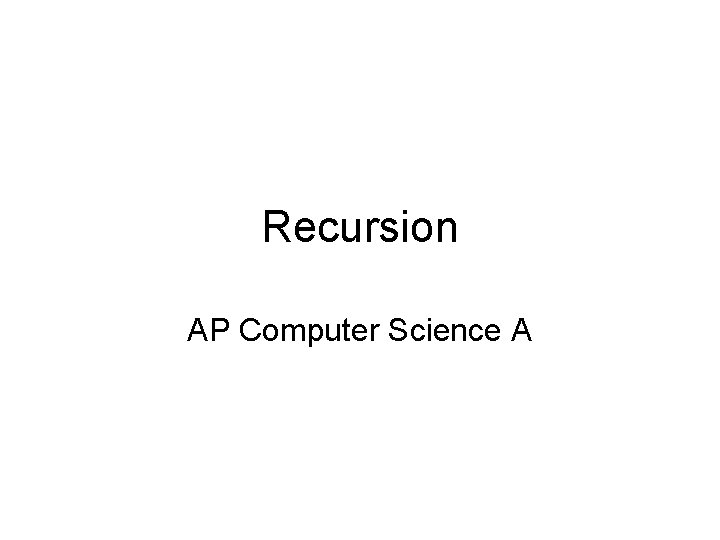Recursion AP Computer Science A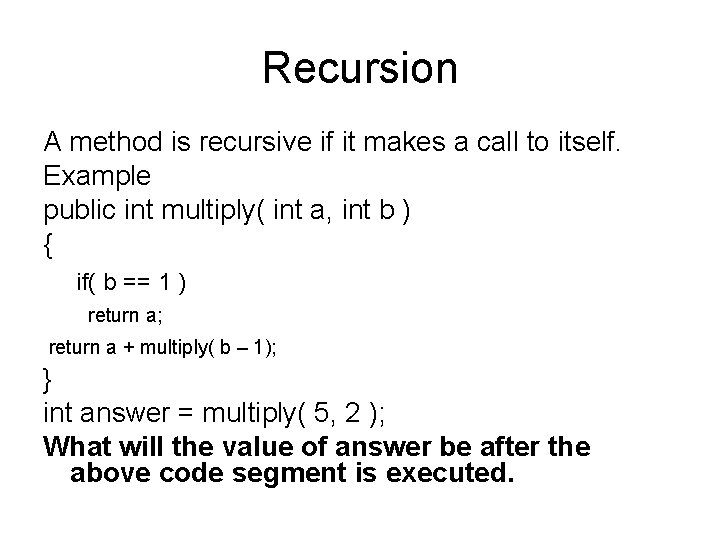Recursion A method is recursive if it makes a call to itself. Example public int multiply( int a, int b ) { if( b == 1 ) return a; return a + multiply( b – 1); } int answer = multiply( 5, 2 ); What will the value of answer be after the above code segment is executed.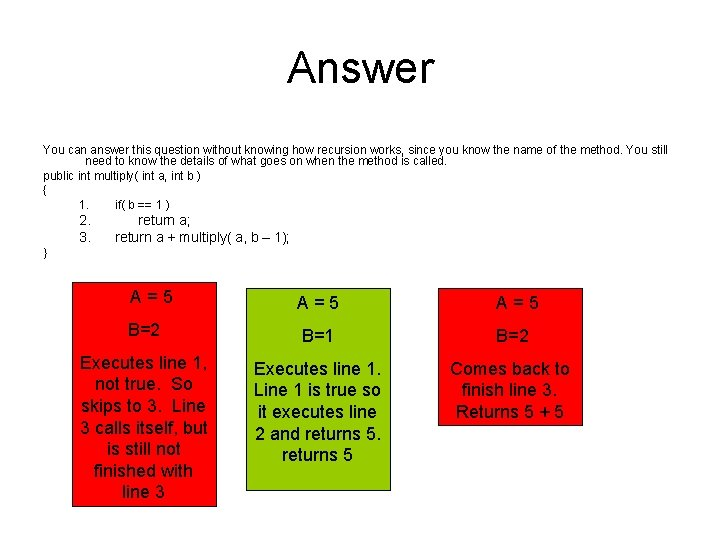Answer You can answer this question without knowing how recursion works, since you know the name of the method. You still need to know the details of what goes on when the method is called. public int multiply( int a, int b ) { 1. if( b == 1 ) 2. 3. return a; return a + multiply( a, b – 1); } A=5 A=5 B=2 B=1 B=2 Executes line 1, not true. So skips to 3. Line 3 calls itself, but is still not finished with line 3 Executes line 1. Line 1 is true so it executes line 2 and returns 5 Comes back to finish line 3. Returns 5 + 5What is the result of the method call mystery( 4 ); ? public void mystery( b ) { if( b == 1) return; for( int i= 0; i < b; i++ ) { System. out. print( “\$”); } System. out. println(); mystery( b – 1 ); }b=4 Answer \$\$\$\$ \$\$ public void mystery( b ) { 1. if( b == 1) 2. return; 3. for( int i= 0; i < b; i++ ) 1 { 2 System. out. print( “\$”); 3 } 4 System. out. println(); 5 mystery( b – 1 ); } b=3 b=2 Executes line 1, not true. So skips to 3. Lines 3 to 6 is a for loop which will print 3 \$ will print 2 \$ will print 4 \$ since b = 2. since b = 4. Then goes to line 7 which prints a line. Then goes to line 8 which calls itself. b = 3. Then goes to line 7 which prints a line. Then goes to line 8 which calls itself. b=1 Executes line 1. It’s true so it returns. Goes back to the other 3 methods that were called. First to the green, then to blue, and finally back to red, and since there is nothing left to do it ends.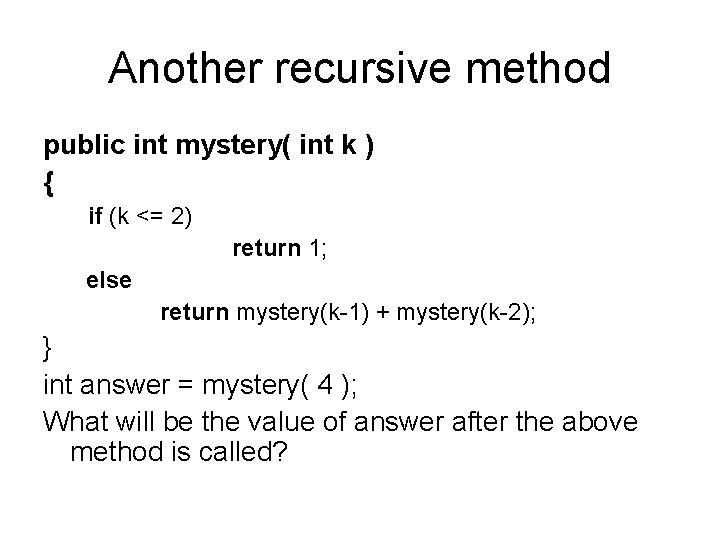Another recursive method public int mystery( int k ) { if (k <= 2) return 1; else return mystery(k-1) + mystery(k-2); } int answer = mystery( 4 ); What will be the value of answer after the above method is called?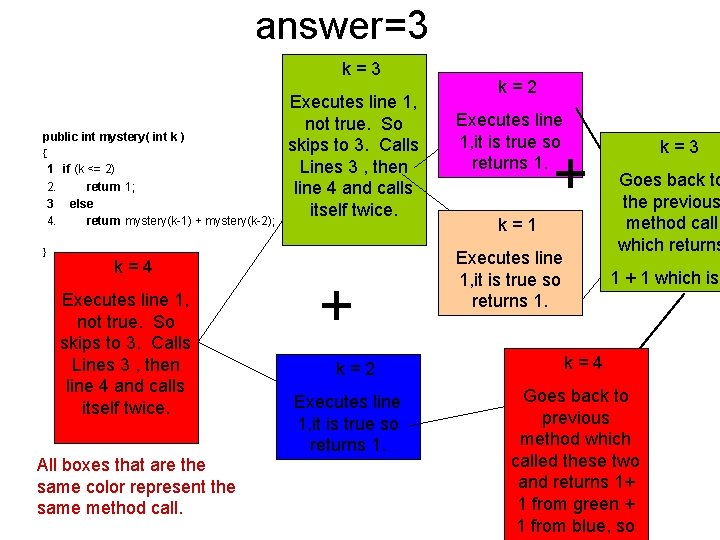answer=3 k=3 public int mystery( int k ) { 1 if (k <= 2) 2. return 1; 3 else 4. return mystery(k-1) + mystery(k-2); } k=4 Executes line 1, not true. So skips to 3. Calls Lines 3 , then line 4 and calls itself twice. All boxes that are the same color represent the same method call. Executes line 1, not true. So skips to 3. Calls Lines 3 , then line 4 and calls itself twice. + k=2 Executes line 1, it is true so returns 1. + k=1 Executes line 1, it is true so returns 1. k=3 Goes back to the previous method call which returns 1 + 1 which is k=4 Goes back to previous method which called these two and returns 1+ 1 from green + 1 from blue, soWhat will the value of mystery( 5 ) be? public int mystery( int k ) { 1 if (k <= 2) 2. return 1; 3 else 4. return mystery(k-1) + mystery(k-2); } int answer = mystery( 5 ); What value will answer have after the above code is executed?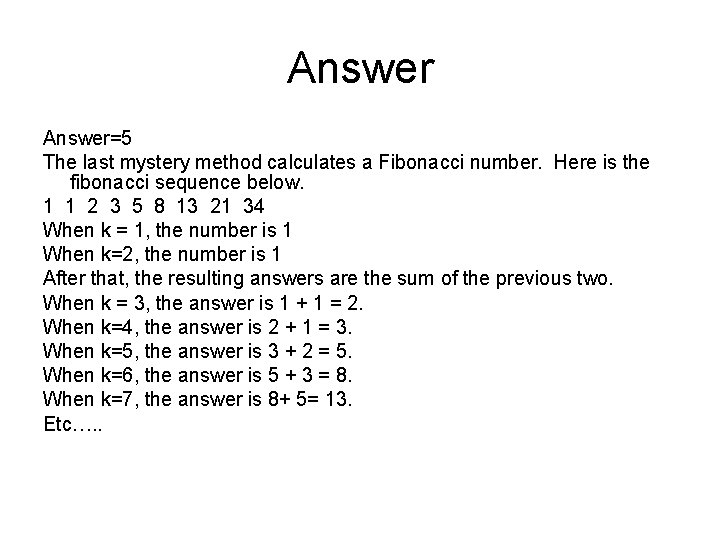Answer=5 The last mystery method calculates a Fibonacci number. Here is the fibonacci sequence below. 1 1 2 3 5 8 13 21 34 When k = 1, the number is 1 When k=2, the number is 1 After that, the resulting answers are the sum of the previous two. When k = 3, the answer is 1 + 1 = 2. When k=4, the answer is 2 + 1 = 3. When k=5, the answer is 3 + 2 = 5. When k=6, the answer is 5 + 3 = 8. When k=7, the answer is 8+ 5= 13. Etc…. .That’s all folks Good Luck Tomorrow!!